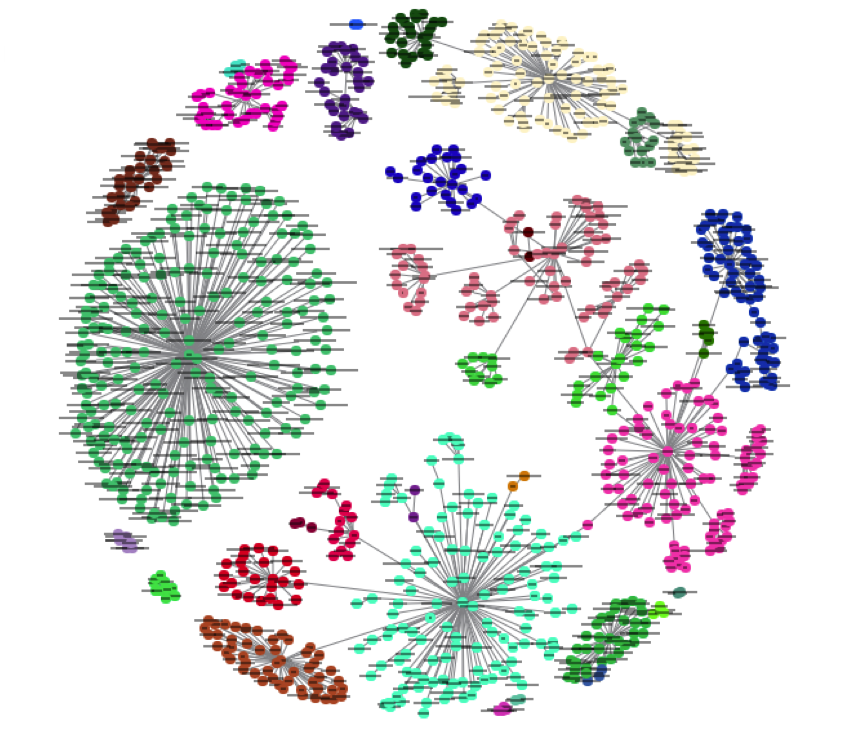# A Practical Introduction to K-Nearest Neighbors Algorithm for Regression (with Python code)

## Introduction

Out of all the machine learning algorithms I have come across, KNN has easily been the simplest to pick up. Despite it’s simplicity, it has proven to be incredibly effective at certain tasks (as you will see in this article).

And even better? It can be used for both classification and regression problems! It’s far more popularly used for classification problems, however. I have seldom seen KNN being implemented on any regression task. My aim here is to illustrate and emphasize how KNN can be equally effective when the target variable is continuous in nature.In this article, we will first understand the intuition behind KNN algorithms, look at the different ways to calculate distances between points, and then finally implement the algorithm in Python on the Big Mart Sales dataset. Let’s go!

1. A simple example to understand the intuition behind KNN
2. How does the KNN algorithm work?
3. Methods of calculating distance between points
4. How to choose the k factor?
5. Working on a dataset

Available here

# K-Nearest Neighbors Demo

This interactive demo lets you explore the K-Nearest Neighbors algorithm for classification.

Each point in the plane is colored with the class that would be assigned to it using the K-Nearest Neighbors algorithm. Points for which the K-Nearest Neighbor algorithm results in a tie are colored white.

You can move points around by clicking and dragging!

Try it here.

More free books and resources,  here

Views: 754

Tags: resource

Comment

Join Data Science Central